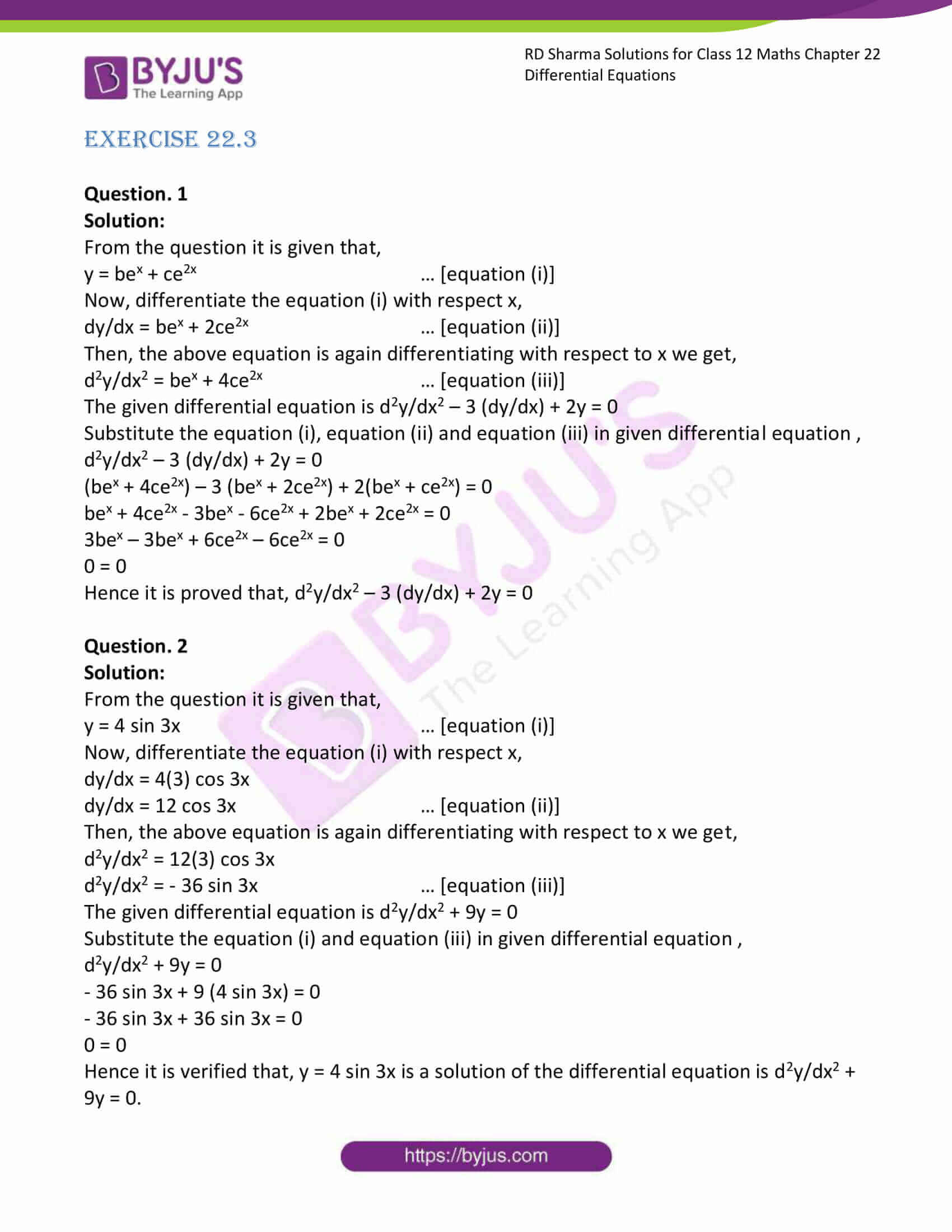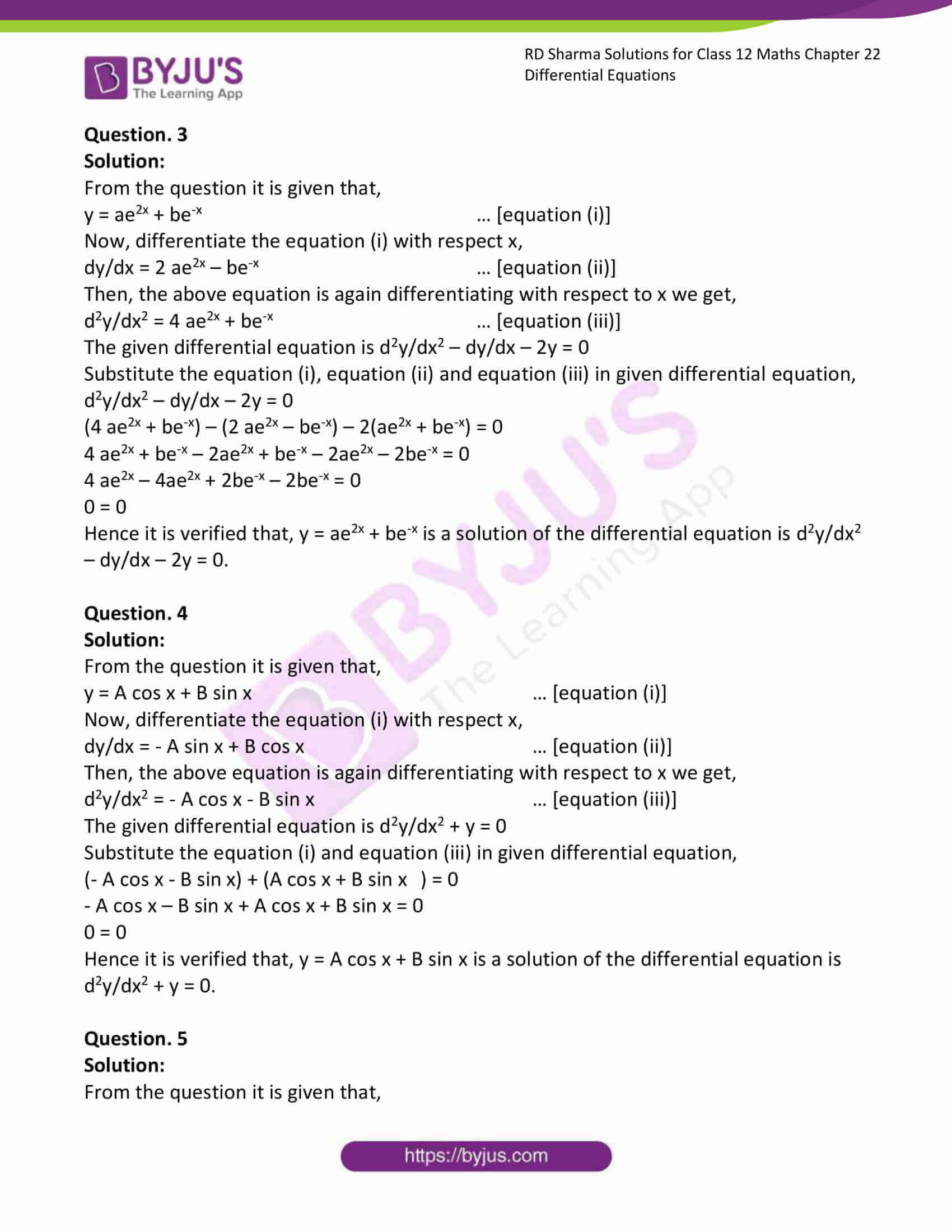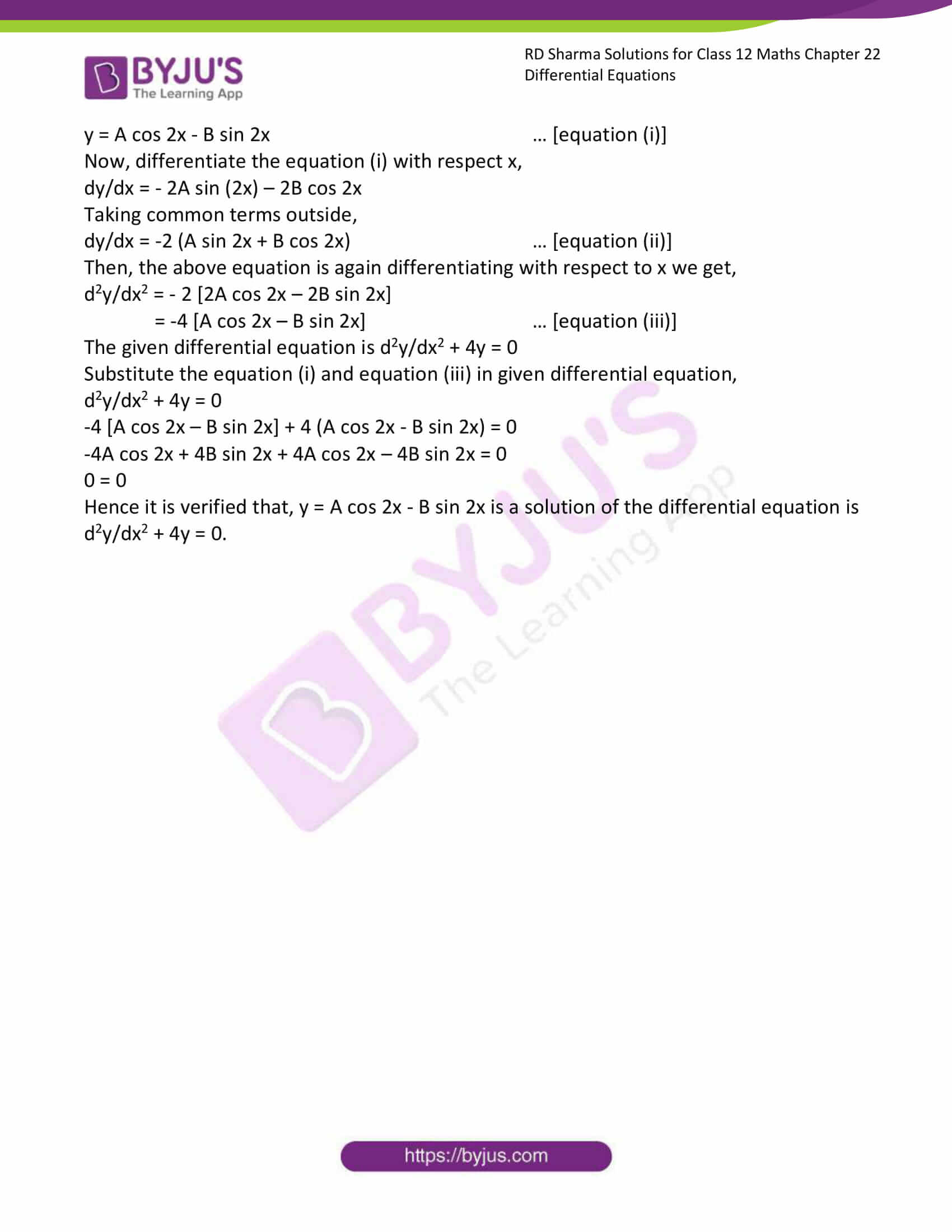# RD Sharma Solutions Class 12 Differential Equations Exercise 22.3

RD Sharma Solutions for Class 12 Maths Exercise 22.3 Chapter 22 Differential Equations are most valuable that assist students in learning complex and challenging topics. These solutions serve as an important study tool when it comes to preparing for the exam. Hence, students are suggested to solve all the questions provided in the RD Sharma Solutions for Class 12 Maths Chapter 22.

## Download PDF of RD Sharma Solutions for Class 12 Maths Chapter 22 Exercise 3### Access RD Sharma Solutions for Class 12 Maths Chapter 22 Exercise 3

EXERCISE 22.3

Question. 1

Solution:

From the question it is given that,

y = bex + ce2x … [equation (i)]

Now, differentiate the equation (i) with respect x,

dy/dx = bex + 2ce2x … [equation (ii)]

Then, the above equation is again differentiating with respect to x we get,

d2y/dx2 = bex + 4ce2x … [equation (iii)]

The given differential equation is d2y/dx2 – 3 (dy/dx) + 2y = 0

Substitute the equation (i), equation (ii) and equation (iii) in given differential equation ,

d2y/dx2 – 3 (dy/dx) + 2y = 0

(bex + 4ce2x) – 3 (bex + 2ce2x) + 2(bex + ce2x) = 0

bex + 4ce2x – 3bex – 6ce2x + 2bex + 2ce2x = 0

3bex – 3bex + 6ce2x – 6ce2x = 0

0 = 0

Hence it is proved that, d2y/dx2 – 3 (dy/dx) + 2y = 0

Question. 2

Solution:

From the question it is given that,

y = 4 sin 3x … [equation (i)]

Now, differentiate the equation (i) with respect x,

dy/dx = 4(3) cos 3x

dy/dx = 12 cos 3x … [equation (ii)]

Then, the above equation is again differentiating with respect to x we get,

d2y/dx2 = 12(3) cos 3x

d2y/dx2 = – 36 sin 3x … [equation (iii)]

The given differential equation is d2y/dx2 + 9y = 0

Substitute the equation (i) and equation (iii) in given differential equation ,

d2y/dx2 + 9y = 0

– 36 sin 3x + 9 (4 sin 3x) = 0

– 36 sin 3x + 36 sin 3x = 0

0 = 0

Hence it is verified that, y = 4 sin 3x is a solution of the differential equation is d2y/dx2 + 9y = 0.

Question. 3

Solution:

From the question it is given that,

y = ae2x + be-x … [equation (i)]

Now, differentiate the equation (i) with respect x,

dy/dx = 2 ae2x – be-x … [equation (ii)]

Then, the above equation is again differentiating with respect to x we get,

d2y/dx2 = 4 ae2x + be-x … [equation (iii)]

The given differential equation is d2y/dx2 – dy/dx – 2y = 0

Substitute the equation (i), equation (ii) and equation (iii) in given differential equation,

d2y/dx2 – dy/dx – 2y = 0

(4 ae2x + be-x) – (2 ae2x – be-x) – 2(ae2x + be-x) = 0

4 ae2x + be-x – 2ae2x + be-x – 2ae2x – 2be-x = 0

4 ae2x – 4ae2x + 2be-x – 2be-x = 0

0 = 0

Hence it is verified that, y = ae2x + be-x is a solution of the differential equation is d2y/dx2 – dy/dx – 2y = 0.

Question. 4

Solution:

From the question it is given that,

y = A cos x + B sin x … [equation (i)]

Now, differentiate the equation (i) with respect x,

dy/dx = – A sin x + B cos x … [equation (ii)]

Then, the above equation is again differentiating with respect to x we get,

d2y/dx2 = – A cos x – B sin x … [equation (iii)]

The given differential equation is d2y/dx2 + y = 0

Substitute the equation (i) and equation (iii) in given differential equation,

(- A cos x – B sin x) + (A cos x + B sin x ) = 0

– A cos x – B sin x + A cos x + B sin x = 0

0 = 0

Hence it is verified that, y = A cos x + B sin x is a solution of the differential equation is d2y/dx2 + y = 0.

Question. 5

Solution:

From the question it is given that,

y = A cos 2x – B sin 2x … [equation (i)]

Now, differentiate the equation (i) with respect x,

dy/dx = – 2A sin (2x) – 2B cos 2x

Taking common terms outside,

dy/dx = -2 (A sin 2x + B cos 2x) … [equation (ii)]

Then, the above equation is again differentiating with respect to x we get,

d2y/dx2 = – 2 [2A cos 2x – 2B sin 2x]

= -4 [A cos 2x – B sin 2x] … [equation (iii)]

The given differential equation is d2y/dx2 + 4y = 0

Substitute the equation (i) and equation (iii) in given differential equation,

d2y/dx2 + 4y = 0

-4 [A cos 2x – B sin 2x] + 4 (A cos 2x – B sin 2x) = 0

-4A cos 2x + 4B sin 2x + 4A cos 2x – 4B sin 2x = 0

0 = 0

Hence it is verified that, y = A cos 2x – B sin 2x is a solution of the differential equation is d2y/dx2 + 4y = 0.